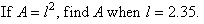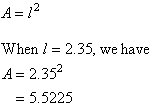Year 9 Interactive Maths - Second Edition

## Formulas

A formula is a rule (or equation) that describes the relationship between variables occurring in some practical situation.

E.g.  A = lw is a formula for the area of a rectangle, A, having length l and width w.

### Substitution

If a formula is given and the values of all but one of the variables in the formula are known, the value of the remaining variable can be evaluated by substituting the known values.

#### Example 33##### Solution: